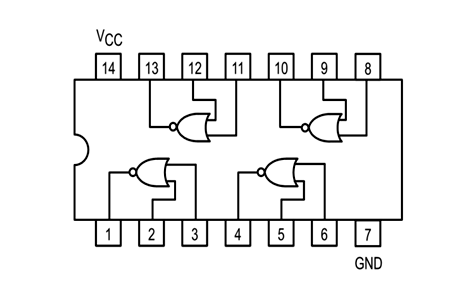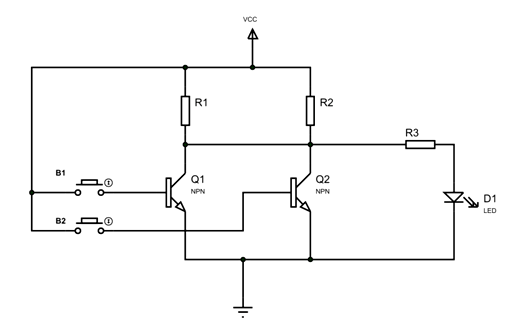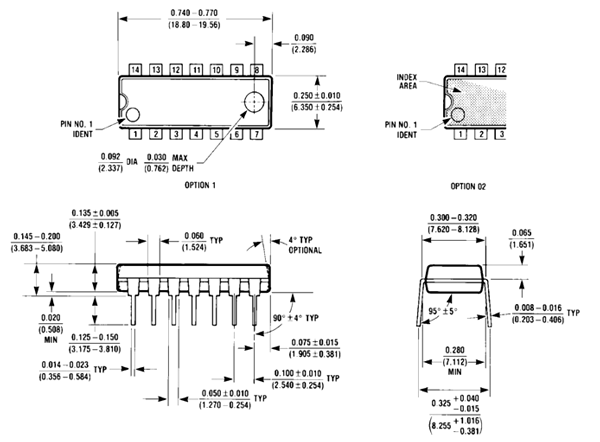# 74LS02 – Quadruple Two Input NOR Gate IC

[Click the image to enlarge it]

74LS02 is a LOGIC GATE IC and member of 74XXYY IC series which are logic gates. There are four NOR gates in the IC and each gate has two inputs, hence the name QUADRUPLE TWO INPUT NOR GATE. The gates in the chip are designed by low power SCHOTTKY TRANSISTORS.

### Pin configuration

74LS02 is a 14 PIN IC as shown in the pinout diagram. The chip is available in different packages and is chosen depending on requirement. The description for each pin is given below.

 Pin Number Description NORGATE 1 2 1A-INPUT1 of GATE 1 3 1B-INPUT2 of GATE 1 1 1Y-OUTPUT of GATE1 NORGATE 2 5 2A-INPUT1 of GATE 2 6 2B-INPUT2 of GATE 2 4 2Y-OUTPUT of GATE2 NORGATE 3 8 3A-INPUT1 of GATE 3 9 3B-INPUT2 of GATE 3 10 3Y-OUTPUT of GATE3 NORGATE 4 11 4A-INPUT1 of GATE 4 12 4B-INPUT2 of GATE 4 13 4Y-OUTPUT of GATE4 SHARED TERMINALS 7 GND- Connected to ground 14 VCC-Connected to positive voltage to provide power to all four gates

### Features and Specifications

• Operating voltage range: +4.75 to +5.25V
• Maximum supply voltage:7V
• Maximum current allowed to draw through each gate output: 8mA
• TTL outputs
• Low power consumption
• Maximum ESD: 3.5KV
• Typical Rise Time: 15ns
• Typical Fall Time: 15ns
• Operating temperature:0°C  to 70°C
• Storage Temperature: -65°C  to 150°C

### 74LS02 Equivalents

SN54LS02, IC 7402, HCF4077, Any two transistors can be reconfigured to form a NOR gate.

### Where to use 74LS02 IC

There are many reasons for using 74LS02. Here are a few cases why it is used.

1. The chipis basically used when (NOR) logic function is required. The chip has four (NOR) gates in it. We can use one or all gates.

2. When you want logic inverter. NOR gates in this chip can be reconnected to make them NOT gates. Each NOR gate can form a single NOT gate. So we can make 74LS02 chip a four NOT gate chip if necessary.

3. Where high speed NOR operation is necessary. As mentioned earlier the gates in the chip are designed bySCHOTTKY TRANSISTORS. With them the switching delays of gates are minimized. Because of this the chip can be used high speed applications.

4. 74LS02 is one of cheapest IC. It is really popular and is available everywhere.

5. The chip also provides TTL outputs which are a must in some systems.

### How to use 74LS02

The four NOR gates in the chip mentioned earlier are connected internally as shown below.Each NOR gate here performs NOR operation for two logic inputs. Also the NOR gate is a combination of OR gate and NOT gate.

So NOR = OR + NOT.

The truth table of NOR gate is given as,

 Input1 Input2 OR Output NOR Output LOW LOW LOW HIGH HIGH LOW HIGH LOW LOW HIGH HIGH LOW HIGH HIGH HIGH LOW

For realizing the above truth table let us take simplified NOR gate and have it connected as shown below.In the circuit two transistors are connected to form a NOR gate. The two inputs are driven out from bases of two transistors. These two inputs are connected to buttons. Output of the gate is taken out from joint collector of both transistors. This output is connected to a LED trough a current limiting resistor. This LED is connected to detect the state of output. The buttons are connected to change the logic of inputs.

Now let us consider a few states:

1. When both buttons are not pressed. In that state the current flow through base of both transistors will be zero.Because base current is zero transistors Q1 and Q2 will be OFF. So the total supply voltage VCC appears across the transistors Q1 and also across Q2. Because output Y1 is nothing but voltage across transistors Q1 or Q2 it will be HIGH. So when INPUTS = LOW, OUTPUT = HIGH.

2. When one of buttons is pressed.Only the relative transistor will be ON leaving the other OFF. The ON transistor will act as short circuit and it will overlap the OFF transistor. With that the total drop across both transistors will be zero. Because transistor drop is zero Y1 will be LOW. So when one INPUT = HIGH, OUTPUT = LOW.

3. When both buttons are pressed. Both transistors will be ON and voltage across both of them will be zero.Because transistor drop is zero Y1 will be LOW. So when both INPUTs = HIGH, OUTPUT = LOW.

After verifying the three states, you can tell that we have satisfied the above truth table.  The output equation for NOR gate can be given as, Y=A+B.

Like this we can use each gate of the chip depending on requirement.

### Applications

• General purpose NOR logic operation
• Digital Electronics
• Servers
• ALUs
• Memory units
• Networking
• Digital systems

### Dimensions

(Inches/millimeters)Component Datasheet